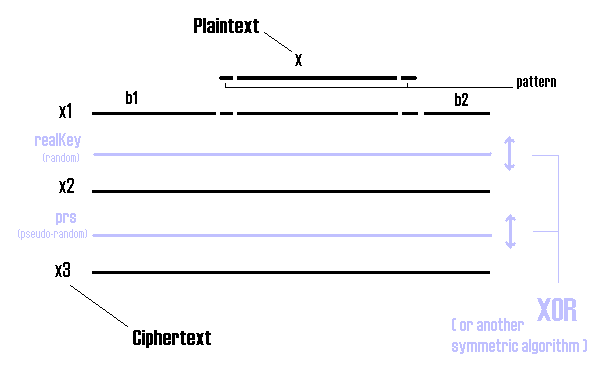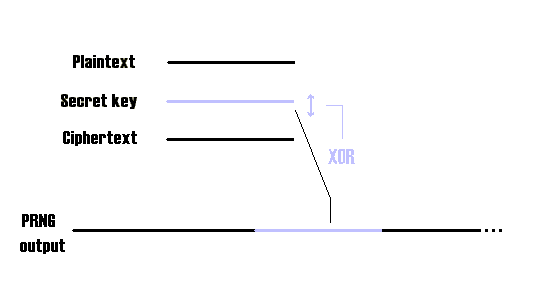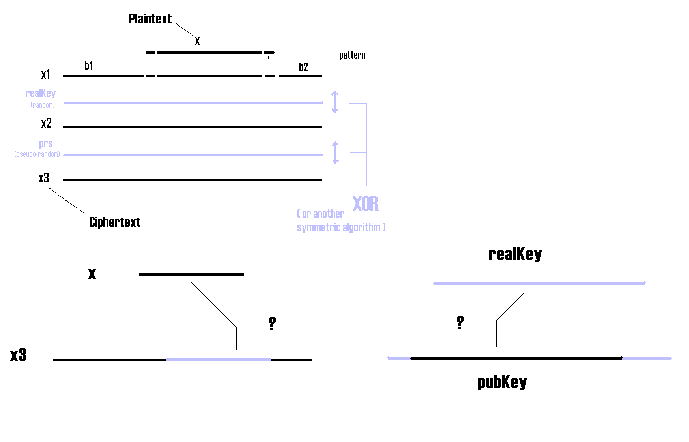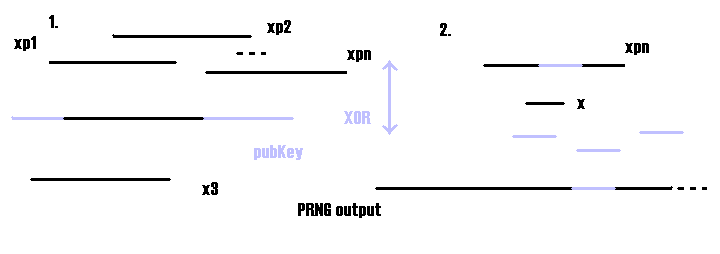Sysepub: Symmetric semi public key algorithm!

Introduction
The algorithms:
- Encryption
- Decryption
Plaintext attack
KPBFA algorithm
Bug List

One time pads (o.t.p) with random key are known to be perfect cryptographical systems. Using a pseudo-random key is more practical, but not perfect anymore. So these apparently very good system have at least 3 big weaknesses or limitations:

- The key must be as long as the plaintext, and sending it may cause the same problems of sending the plaintext (limitation of the random o.t.p)

- The pseudo-random generator is periodic in general (weakness)

- The pseudo-random o.t.p. is subject to the plaintext attacks; if someone gets a portion of plaintext he could find out the key (weakness)

My effort is about trying to eliminate these weaknesses and limitation in the following way.
Encryption is performed in 3 main steps.
Let's call A the sender and B the receiver.
- step 1: The plaintext is prepared for encryption. I add at the beginning and at the end of the byte array of plaintext (let's call it x) a short secret byte array (let's call it pattern), then I add two random arrays of random length (the default in Sysepub is 2*x.length at most). The random arrays add very little computational time for the legitimate users, and they complicate cryptanalysis (cryptanalyst won't know WHERE is the real message within x1) especially in a plaintext attack.
- step 2: Here comes out the "public" key. The byte array obtained in step 1 (x1) is XORed with a random byte array. Why it's public? Because, for example, A could generate it in a truly random way, and send it to B even with the ciphertext! But the real key in step 2 isn't exactly the public array pubKey, that must be longer than x1, but a subset of pubKey. This subset is the array realKey obtained from the elements: pubKey[pr] .. pubKey[pr + x1.length] . The positive integer pr < pubKey.length-x1.length is obtained from a pseudo-random generator whose seed is the secret key shared with B.
- step 3: the ciphertext obtained (x2) is XORed with a pseudo-random sequence (prs) obtained from the same pseudo-random generator (or a new one, but you will need another secret seed).The byte array x3 obtained is the final ciphertext and can be sent with the public key to B. Now let's see how B deciphers it.
- step 1: B deciphers x3 and gets x2, as he has the secret seed and he can generate the pseudo-random sequence.
- step 2: now B can compute pr with the secret seed; hence he can obtain the real key from pubKey, and so obtains x1.
- step 3: B easily obtains x from x1 searching for the secret pattern.

Now I present the algorithm in a more formal way (using pseudo-code).
I guess the following notations are obvious:
!= is "not equals"; byte[] is a byte array; x.length is the length of the byte[] x
XOR between two byte array (of the same length) is equivalent to XORing byte per byte from the first to the last byte.

Suppose that are defined the following functions:
PRBG(seed, n) is a Pseudo Random Byte Generator initialized with the integer seed
(returns a byte array of length n)
PRNG(seed, n) is a Pseudo Random Number Generator initialized with the integer seed
(returns a positive integer smaller than n)
time(t, ms) returns an integer in function of the current time interval (t is the current time in milliseconds from a starting date, ms is the number of milliseconds in the interval)
(e.g.: time(t, 86400000) every 24 hours returns a different integer)

Sysepub algorithm (encryption)

INPUT: The byte arrays x (the plaintext), pubKey (public), pattern (private)
The integers seed (private) and pL, pKL>x1L (the lengths of the arrays)
OUTPUT: The byte array x3 (the ciphertext)

Constants: msecDay = 86400000 (the number of milliseconds in a day)
the integers bound, period (user specified)
Variables: byte[] x1, realKey, prs, b1, b2
integers pr, x1L, n1, n2

SysepubE(x, pubKey, pattern, seed)

n1 = PRNG(time(t,1) , bound) n2 = PRNG(time(t,1) , bound)
b1 = PRBG(time(t,1) , n1) b2 = PRBG(time(t,1) , n2)
x1 = {b1, .. , b1[n1], pattern, .. , pattern[pL], x, .. , x[xL],
pattern, .. , pattern[pL], b2, .. , b2[n2]}
x1L = x1.length
pr = PRNG(seed + time(t , period*msecDay) , pKL - x1L)
realKey = {pubKey[pr], .. , pubKey[pr + x1L]}
prs = PRBG(seed + time(t, period*msecDay), x1L)
x3 = x1 XOR (realKey XOR prs)
return x3

Sysepub algorithm (decryption)

INPUT: The byte arrays x3 (the ciphertext), pubKey (public), pattern (private)
The integers seed (private) and x3L, pKL, pL (the lengths of the arrays)
OUTPUT: The byte array x (the plaintext)

Constant: msecDay = 86400000 (the number of milliseconds in a day)
Variables: byte[] x1, realKey, prs
integer pr
Function: indexOf(pattern, arraySearch, start) returns the index within the array arraySearch of the first occurrence of the specified subarray pattern, starting at the specified index start (-1 if not found).

SysepubD(x, pubKey, pattern, seed)

prs = PRBG(seed + time(t , period*msecDay), x3L)
x2 = x3 XOR prs
pr = PRNG(seed + time(t , period*msecDay), pKL - x1L)
realKey = {pubKey[pr], .. , pubKey[pr + x3L]}
x1 = x2 XOR realKey
first = indexOf(pattern, x1, 0)
if (first != -1)
last = indexOf(pattern, x1, first + 1)
x = {x1[first + pL], .. , x1[last]}
return x
else return null

Why I think this system is innovative.

The limitation of the random o.t.p. is solved: the secret key is in fact very short (the seed and the pattern require few bytes) and must be shared between A and B only one time.
The periodicity of the pseudo-random generator isn't anymore a problem: even if, in time, the same sequence should occur again, the realKey would be different every time and cryptanalisys made more difficult.

The plaintext attack : this is the critical point, where I conjecture that this system is immune to. First of all let's see how it can be successfully made in the simple case of pseudo-random o.t.p.In this case, if an enemy C could get a long enough portion of plaintext with the ciphertext, he could find out with a simple XOR the key generated by the PRNG initialized with the secret seed. The graph shows what C has got to do: knowing what PRNG was used, he could easily generate the pseudo-random output, until the exact sequence of the key is found. Now he could easily decrypt other ciphertext enciphered with the same system: for example if A and B simply used every time the next bytes generated by the PRNG, all that C has to do is to take the next ciphertext and, eventually making some shifts, XORing with the next pseudo-random bytes. That's why pseudo-random o.t.p are risky.
Now, let's see why I believe Sysepub is much more strong against plaintext attacks.Suppose that C has one, or more, pairs of plaintext/ciphertext (x,x3). Now, suppose also that he knows pubKey: he could XOR x3 with every possible subset of length x3.length. With pubKey.length - x3.length = 500 K this means 500000 possible texts xp that, knowing the secret seed, would lead to x. Now what kind of cryptanalysis C could do? That is the question. I conjecture that all the, say 500000 xp, have the same entropy, coming from the pseudo-random sequence x3 (x1 XOR realKey XOR prs), XORed with 500000 truly random sequencies.C could take a xp and XOR it with x to try obtaining the prs: but xp is longer than x, so he should try every subset of xp of the length of x; and so on, for every xp obtained. Moreover C could even obtain multiple sequences compatible with the PRNG output. In conclusion the computational time is much greater than the simple case of pseudo-random o.t.p.
I wrote, using pseudo-code, an algorithm to try the plaintext attack: its (ugly) name is KPBFA (Known Plaintext Brute Force Attack).
This algorithm tries to do, with a brute force approach, the steps described above to find the seed knowing a pair of plaintext/ciphertext.

KPBFA algorithm

INPUT: The byte arrays x (the plaintext), x3 (the ciphertext), pubKey
and their lengths (integers xL, x3L, pKL)

Variables: byte[] subx3, subpk, xor1, xor2, plain
Functions: subset(a,b) is an algorithm that checks if b is a subset of a (returns true or false)
SSP# is a modified version of SysepubD (decryption) that works without knowing the secret pattern (it can only return x1, not x)
BFS(prs) is the algorithm that returns the seed that generates the p.r. sequence prs with the PRBG function (-1 if doesn't exist).

KPBFA(x, x3, pubKey)

1. d1 = pKL - x3L ; d2 = x3L - xL
2. For i = 0 to d1 do the following:
2.1 subpk = {pubKey[i], ... , pubKey[i + x3L]}
2.2 xor1 = x3 XOR subpk
2.3 For j = 0 to d2 do the following:
2.3.1 subxor1 = {xor1[j], xor1[j + 1], ... , xor1[j + xL]}
2.3.2 xor2 = subxor1 XOR x
2.3.3 seed = BFS(xor2)
2.3.4 if (seed!=-1)
2.3.4.1 plain = SSP#(seed, x3, pubKey)
2.3.4.2 if (subset(plain,x)) return seed
3. Return -1

To make an evaluation I'll refer to the
Sysepub challenge example. Let's call t the time needed to perform the steps from 2.3.1 to 2.3.4. In the challenge the plaintext is of 56 KB, the ciphertext of 194 KB and the pubKey of 200 KB. So there are nearly 6000 possible xps, and for each of them, if he is unlucky, C must try nearly 138000 subsets of the length of x. In other words d1~6000, d2~138000 Then the computational time is nearly d1*d2*t~6,000*138,000*t = 828,000,000*t in the worst case.

I made a statistical test using the ent program (http://www.fourmilab.ch/random/) using a plaintext of 315 K identical bytes; Sysepub enciphered it into a 829KB file. The results were very good: optimum compression, 0% ; mean value 127.6334 (127.5 = random); Monte Carlo value for Pi is 3.141996862 (error 0.01 percent); Serial correlation coefficient is -0.000079 (totally uncorrelated = 0.0); normal Chi square distribution.

Few words about the Java implementation: it's called Sysepub (acronymous of Symmetric Semi Public ..). As random generator I used the java.util.Random class, probably not really a CSPRNG, but it can be easily substituted by a more cryptographically strong generator in the A1timeP class (the class used as one time pad key generator).

Bug List
The alpha test finally produced a bad guy! It is caused by the differencies between the Operating Systems.
The bug happened attempting to decipher a message on a Windows platform, after enciphering it on a Linux platform. I guess that the two OS may interpret differently some bytes in a file, and since the length of the ciphertext must be exactly the same in encryption and decryption, I think that very few bytes has compromised the decryption of several KB. Anyway, usually decryption works properly even between Linux and Windows.
I will try to fix this (if you have an idea your suggestions are welcome).
However this bug seems not to happen among different Windows versions (if you have experienced other cases, send me you reports!).
Send ideas and reports using
this form.